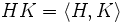# Product of subgroups

## Definition

### Symbol-free definition

The Product of two subgroups of a group is the subset consiting of the paarwise products between the two subgroups.

### Definition with symbols

The Product$HK$ of two subgroups$H$ and$K$ of a group$G$ is:$HK := \{ hk \mid h \in H, k \in K \}$.

If$G$ is abelian and if the group operation is denoted as$+$, the product is termed the sum, and is denoted$H + K$:$H + K = \{ h + k \mid h \in H, k \in K \}$.

## Facts$HK$ is the double coset$HeK$,$e$ being the identity element of$G$.

The cardinality$\left| HK \right|$ of$HK$ is$\left| H \right| \left| K \right| / \left| H \cap K \right|$.

The product$HK$ is in general not a subgroup, because it may not be closed under the group operation.

The smallest subgroup containing$HK$ is the join$\langle H, K \rangle$ of$H$ and$K$, which is also the subgroup generated by$H$ and$K$.

Following statements are equivalent:

•$HK$ is a subgroup
•$HK = \langle H,K \rangle$, viz., it is precisely the join of$H$ and$K$ (the subgroup generated by$H$ and$K$)
•$\! HK = KH$
•$HK \subseteq KH$
•$KH \subseteq HK$

If the above equivalent conditions hold,$H$ and$K$ are termed permuting subgroups.